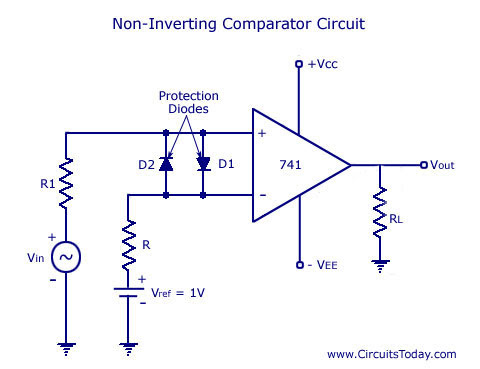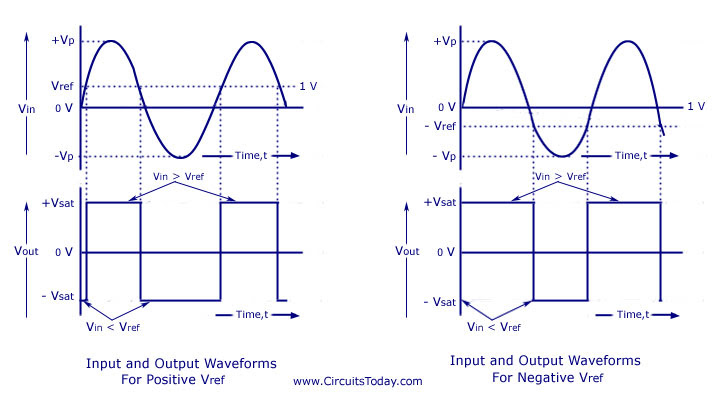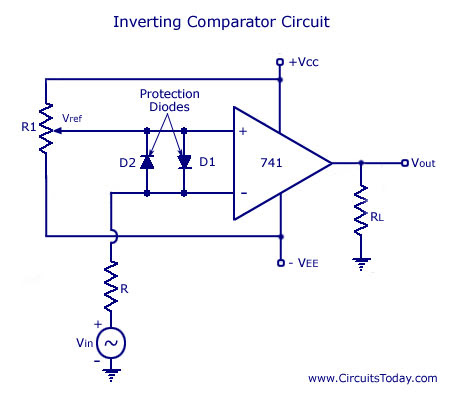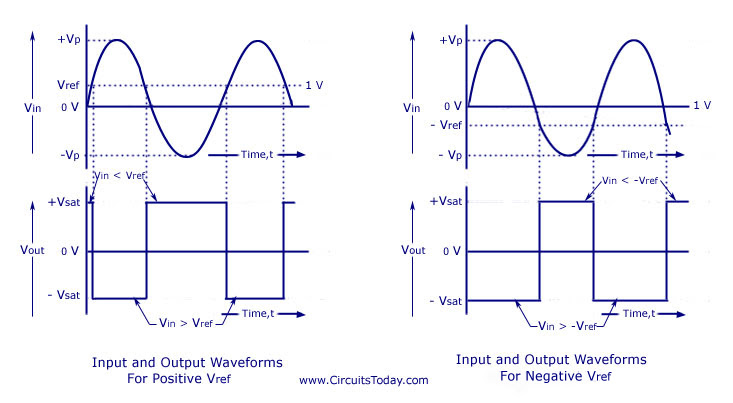## Audio

[Audio][slideshow]

In this post we will be discussing about the op-amp as a comparator.We have already discussed other applications of the op-amp in rectangular wave form generator circuits like astable (or free-running) multivibrators, monostable multivibrators (or one-shot) and bistable multivibrators (or flip-flops).

To get a better understanding of operational amplifiers click here:- Operational Amplifiers (Op-amp)

## Op-amp Comparator

A comparator finds its importance in circuits where two voltage signals are to be compared and to be distinguished on which is stronger. A comparator is also an important circuit in the design of non-sinusoidal waveform generators as relaxation oscillators.

In an op-amp with an open loop configuration with a differential or single input signal has a value greater than 0, the high gain which goes to infinity drives the output of the op-amp into saturation. Thus, an op-amp operating in open loop configuration will have an output that goes to positive saturation or negative saturation level or switch between positive and negative saturation levels and thus clips the output above these levels. This principle is used in a comparator circuit with two inputs and an output. The 2 inputs, out of which one is a reference voltage (Vref) is compared with each other.

## Working of 741 IC Op-amp Comparator Circuit

### Non-inverting 741 IC Op-amp Comparator Circuit

A non-inverting 741 IC op-amp comparator circuit is shown in the figure below. It is called a non-inverting comparator circuit as the sinusoidal input signal Vin is applied to the non-inverting terminal. The fixed reference voltage Vref is give to the inverting terminal (-) of the op-amp.

When the value of the input voltage Vin is greater than the reference voltage Vref the output voltage Vo goes to positive saturation. This is because the voltage at the non-inverting input is greater than the voltage at the inverting input.741 IC Non-Inverting Comparator Circuit

When the value of the input voltage Vin is lesser than the reference voltage Vref, the output voltage Vo goes to negative saturation. This is because the voltage at the non-inverting input is smaller than the voltage at the inverting input. Thus, output voltage Vo changes from positive saturation point to negative saturation point whenever the difference between Vin and Vref changes. This is shown in the waveform below. The comparator can be called a voltage level detector, as for a fixed value of Vref, the voltage level of Vin can be detected.

The circuit diagram shows the diodes D1and D2. These two diodes are used to protect the op-amp from damage due to increase in input voltage. Thes diodes are called clamp diodes as they clamp the differential input voltages to either 0.7V or -0.7V. Most op-amps do not need clamp diodes as most of them already have built in protection. Resistance R1 is connected in series with input voltage Vin and R is connected between the inverting input and reference voltage Vref. R1 limits the current through the clamp diodes and R reduces the offset problem.741 IC Op-Amp Non-Inverting Comparator Waveform

### Inverting 741 IC Op-amp Comparator Circuit

An inverting 741 IC op-amp comparator circuit is shown in the figure below. It is called a inverting comparator circuit as the sinusoidal input signal Vin is applied to the inverting terminal. The fixed reference voltage Vref is give to the non-inverting terminal (+) of the op-amp. A potentiometer is used as a voltage divider circuit to obtain the reference voltage in the non-inverting input terminal. Bothe ends of the POT are connected to the dc supply voltage +VCC and -VEE. The wiper is connected to the non-inverting input terminal. When the wiper is rotated to a value near +VCC, Vref becomes more positive, and when the wiper is rotated towards -VEE, the value of Vref becomes more negative. The waveforms are shown below.Op-amp 741 IC Inverting Comparator Circuit741 IC Op-Amp Inverting Comparator Waveform

### Comparator Characteristics

1. Operation Speed – According to change of conditions in the input, a comparator circuit switches at a good speed beween the saturation levels and the response is instantaneous.

2. Accuracy – Accuracy of the comparator circuit causes the following characteristics:-

(a) High Voltage Gain – The comparator circuit is said to have a high voltage gain characteristic that results in the requirement of smaller hysteresis voltage. As a result the comparator output voltage switches between the upper and lower saturation levels.

(b) High Common Mode Rejection Ratio (CMRR) – The common mode input voltage parameters such a noise is rejcted with the help of a high CMRR.

(c) Very Small Input Offset Current and Input Offset Voltage – A negligible amount of Input Offset Current and Input Offset Voltage causes a lesser amount of offset problems. To reduce further offset problems, offset voltage compensating networks and offset minimizing resistors can be used.

[Lights][grids]